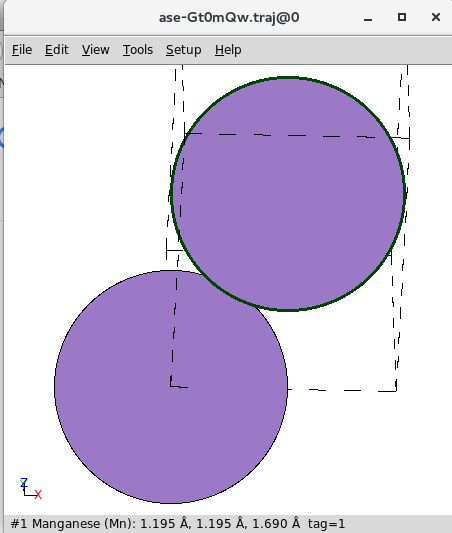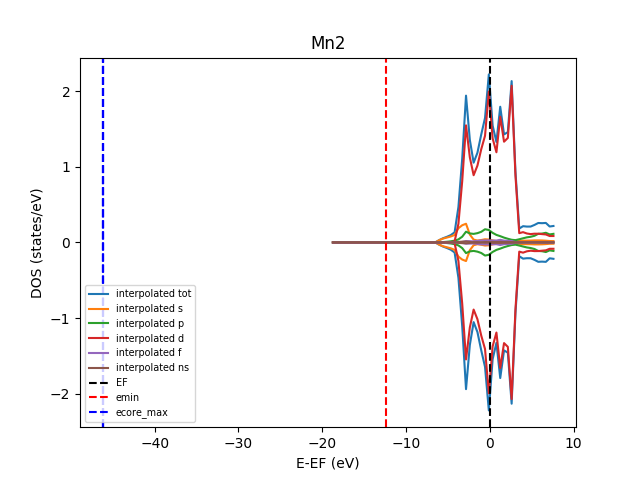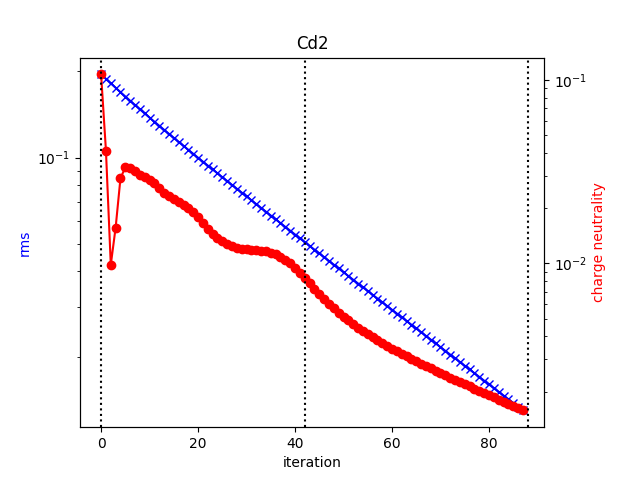# Tools

Here the tools provided by `aiida-kkr` are described.

## Plotting tools

Visualize typical nodes using `plot_kkr` from `aiida_kkr.tools.plot_kkr`. The `plot_kkr` function takes a node reference (can be a pk, uuid or the node itself or a list of these) and creates common plots for a quick visualization of the results obtained with the `aiida-kkr` plugin.

Usage example:

```from aiida_kkr.tools.plot_kkr import plot_kkr
# use pk:
plot_kkr(999999)
# use uuid:
plot_kkr('xxxxx-xxxxx')
# used actual aiida node:
# give list of nodes which goups plots together
```

The behavior of `plot_kkr` can be controled using keyword arguments:

```plot_kkr(99999, strucplot=False) # do not call ase`s view function to visualize structure
plot_kkr(99999, silent=True) # plots only (no printout of inputs/outputs to node)
```

List of `plot_kkr` specific keyword arguments:

• `silent` (bool, default: `False`): print information about input node including inputs and outputs

• `strucplot` (bool, default: `True`): plot structure using ase’s `view` function

• `interpol` (bool, default: `True`): use interpolated data for DOS plots

• `all_atoms` (bool, default: `False`): plot all atoms in DOS plots (default: plot total DOS only)

• `l_channels` (bool, default: `True`): plot l-channels in addition to total DOS

• `logscale` (bool, default: `True`): plot rms and charge neutrality curves on a log-scale

Other keyword arguments are passed onto plotting functions, e.g. to modify line properties etc. (see matplotlib documentation for a reference of possible keywords to modify line properties):

```plot_kkr(99999, marker='o', color='r') # red lines with 'o' markers
```

### Examples

#### Plot structure nodeVisualize a structure node (also happens as sub-parts of workflows that have a structure as input if strucplot is not set to False). Shown is a screenshot of the ouput produced by ase’s view.

#### Plot output of `kkr_startpot_wc` workflowVisualize the output of a kkr_startpot_wc workflow. The starting DOS is shown and the vertical lines indicate the position of the highest core states, the start of the ernergy contour and the Fermi level.

#### Plot output of `kkr_scf_wc` workflowVisualize the output of an unfinished kkr_scf_wc workflow. The vertical lines indicate where individual calculations have started and ended.

#### Plot output of `kkr_eos_wc` workflow

```plot_kkr([34157,31962, 31974], silet=True, strucplot=False, logscale=False)